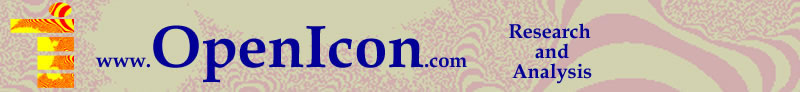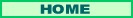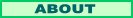µ Machine Understanding

Search

More Tech Stuff:

Indexing Books: Lessons in Language Computations

Client-Side Frame Manipulation Inside the Microsoft Internet Explorer Object Model with Visual Basic .NET

Replacing a PC power supply

Constructing a Mandelbrot Set Based Logo with Visual Basic.NET and Fireworks

March 4, 2010

Evaluating HTMs, Part 3: How do HTMs Learn?

"Hierarchical Temporary Memory, Concepts, Theory, and Terminology " by Hawkins and George, Section 2, "How Do HTMs Discover and Infer Causes", gives an overview of the internal mechanisms of HTMs.

Specifically, it gives an a overview of how HTMs learn. This prompted me to think about the difference between learning and discovery. They could be the same thing, but learning for humans often implies a teacher presenting information to be learned to a student. Discovery implies coming upon something new (perhaps a relationship between already known objects) and realizing that it is new and should be remembered.

Each node in an HTM uses the same algorithm. The nodes are arranged hiearchically, with representations typically showing a row of nodes at the bottom that take input. Each row above the bottom has progressively fewer nodes. The top node is a single node. Its output is a vector that represents a cause, or object related to the data in a causal fashion. In fact each node does this, passing its output vector to the next higher row of nodes. So causes are built up hierarchically. All data and discoveries include a time element. In a visual field, for instance, the time element could be no change in a part of the field, or changing color with time, or following a spot of color from one part of the visual field to another over a course of time.

Get used to the technical use of the term "belief" if you want to follow discussions about HTMs. This term is used extensively in probabilistic reasoning theory. "A belief is an internal state of each node," but it does correspond to a probability that there is a causal relationship in the data. "I believe the lion must have escaped from the zoo," is a sentence that conveys to us that a person lives where lions do not live in the wild; it differs from "I know ..." because the speaker is admitting there are other possible causes. In a simple HTM, in a lower node, a belief might be something like "28% probability that this is a horizontal line, 16% that this is two animal eyes, etc." Again, beliefs are represented in software by vectors, but they are not generally identical to the output vectors of the nodes.

In training or learning, the HTM forms new beliefs at the bottom of the hierarchy of nodes first. More complex beliefs can only be created once lower level beliefs exist, but the entire process is flexible. If a lower level node alters its belief, it tends to effect higher level nodes. So learning is not just memorization.

So how does a node do all this? Nodes are given a set number of "quantization points." Here the authors are not very clear. The input pattern is assigned to one of the quantization points. And/or "the node decides how close (spacially) the current input is to each of the quantization points and assigns a probablity to each quantization point." How it decides is presumably an algorithm. With enough quantization points, each input data set could be matched exactly to a point. Would that set up cause the node to fail? To do as the authors say, the assumption is there are less quantization points than there are possible inputs.

Step two is "the node looks for common sequences of these quantization points" and "represents each sequence with a variable." So you have to ask, why assign probabilities, why not just assign closest fits? In any case the output variable represents a sequence of quantization points based on the sequence of input data.

Admitting that the authors are introducing the topic, and its vocabulary, still I would have liked more than two short paragraphs on the internal operations of HTM nodes.

Interestingly (and copying what is known about the cortex of mammal brains) information can move both up and down the hierarchy of nodes. As just described, data moving up the hierarchy is temporal variables. Data going down the hierarchy represents the "distribution over the quantization points." That would be a probability distribution.

What I suspect the authors mean is that there is a mechanism to alter the quantization points themselves. Points with long-term zero percent probabilities don't help resolve ambiguity. The set of point probabilities being sent down the hierarchy allows the (lower) node to "take a relatively stable pattern from its parent node(s)" and "turn it into a sequence of spatial patterns."

The claim is that over time "patterns move from the bottom of the hierarchy to the top." In effect rather than sending the raw data up the hierarchy, the nodes send "names" of data sequences up the hierarchy.

That would be pretty cool, and I'd like to know exactly how it happens, but this is, afterall, only an introduction.